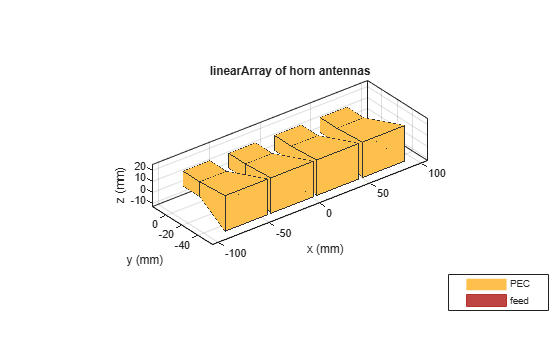# Analyze Cylindrical Reflector Antenna with Horn Array Feed

This example shows how to analyze an antenna consisting of a cylindrical reflector with a linear array of horns.

### Create Horn Antenna

Create a horn antenna resonating at 10 GHz. Set `lambda` to the free space wavelength at the design frequency of 10 GHz.

```freq = 10e9; lambda = physconst('light')/freq; h = design(horn,10e9); h.FlareWidth = 0.0416; h.FlareLength = 0.0416; h.FlareHeight = 31.8e-3; h.Length = 21e-3; h.FeedOffset(1) = -3.6e-3; figure; show(h)```### Plot VSWR and Radiation Pattern of Horn Antenna

Plot the voltage standing wave ratio (VSWR) of the horn antenna over the frequency range of 8.5–12.5 GHz.

```range = (0.85:0.01:1.25)*freq; figure; vswr(h,range); ylim([0 5]);```The VSWR of the horn antenna is less than 2 between 8.6–12.2 GHz.

Plot the radiation pattern of the horn antenna.

```figure; pattern(h,freq);```The directivity of the antenna is 11.4 dB.

### Create Linear Array of Horns

Create a linear array of horns. Set the number of elements in the array to 4 and the spacing between the elements to 1.5 times of lambda.

```arr = linearArray('Element',h,'NumElements',4); arr.Element.Tilt = -90; arr.Element.TiltAxis = 'Z'; arr.ElementSpacing = 1.5*lambda; figure; show(arr)```### Create Cylindrical Reflector with Horn Array

Create a cylindrical reflector with the linear array of horns as the exciter. Tilt the exciter at an angle of 90 degrees along the Z-axis and 70 degrees along the Y-axis.

```ref = reflectorCylindrical; ref.GroundPlaneLength = 10.2*lambda; ref.GroundPlaneWidth = 10.3*lambda; ref.Spacing = 8.5*lambda; ref.Tilt = 90; ref.Exciter = arr; ref.Exciter.Tilt = [90 70]; ref.Exciter.TiltAxis = ['Z','Y']; figure; show(ref)```### Mesh and Plot Radiation Pattern of Cylindrical Reflector with Horn Array

Mesh the reflector manually with the maximum edge length of `lambda/10`.

```figure; mesh(ref,'MaxEdgeLength',lambda/10);```Load the solved reflector file `refObj.mat`.

`load("refObj.mat");`

```figure; pattern(ref,freq); view([80 10]);```You can also plot the radiation pattern without using the MAT file, but the `pattern(ref,freq)` command takes up to 18 minutes to plot the radiation pattern. To run the `pattern(ref,freq)` command, these machine configurations are preferred:

• Processor: Intel ® Xeon ® CPU E5-1650 v4 @3.60GHz.

• RAM: 64GB.

• System type: 64-bit operating system.

The directivity of the cylindrical reflector antenna with a horn array feed is 18.7 dB.